## Circular Motion and Gravitation Review

#### Navigate to:

 [ #1 | #2 | #3 | #4 | #5 | #6 | #7 | #8 | #9 | #10 | #11 | #12 | #13 | #14 | #15 | #16 | #17 | #18 | #19 | #20 | #21 | #22 | #23 | #24 | #25 | #26 | #27 | #28 | #29 | #30 | #31 | #32 | #33 | #34 | #35 | #36 | #37 | #38 | #39 | #40 ]

### Part A: Multiple Choice

1. Which of the following statements are true of an object moving in a circle at a constant speed? Include all that apply.

1. The object experiences a force which has a component directed parallel to the direction of motion.
2. Inertia causes objects to move in a circle.
3. There can be a force pushing outwards on the object as long as the net force in inwards.
4. Because the speed is constant, the acceleration is zero.
5. The acceleration and the net force vector are directed perpendicular to each other.
6. If the net force acting upon the object is suddenly reduced to zero, then the object would suddenly depart from its circular path and travel tangent to the circle.
7. The acceleration of the object is directed tangent to the circle.

 Answer: CF A is false; if the motion is in a circle at constant speed, the net force is perpendicular to the direction of motion and there is neither a component parallel nor anti-parallel to the direction of motion.) B is false; it is centripetal force which causes the circular motion. Inertia (which is NOT a force) is merely the tendency of any moving object to continue in its straight-line constant speed path. C is true; an object which moves in a circle must have a net inward force. There are many instances of individual outwards forces which are exceeded by an individual inward force (e.g., see #5 below). D is false; acceleration occurs when there is a change in velocity. Since the direction of the velocity vector is changing, there is an acceleration - an inward acceleration. E is false; the acceleration and net force are always directed in the same direction. In this case, F and a are directed inward; this happens to be perpendicular to the tangential velocity vector. F is true; if the net force is 0 N, then the moving object will maintain its state of motion. At the instant that Fnet becomes 0 N, the object is moving tangent to the circle. G is false; the acceleration is directed inwards; only the velocity is directed tangent to the circle.

 Useful Web Links Speed and Velocity || Acceleration || The Centripetal Force Requirement

[ #1 | #2 | #3 | #4 | #5 | #6 | #7 | #8 | #9 | #10 | #11 | #12 | #13 | #14 | #15 | #16 | #17 | #18 | #19 | #20 | #21 | #22 | #23 | #24 | #25 | #26 | #27 | #28 | #29 | #30 | #31 | #32 | #33 | #34 | #35 | #36 | #37 | #38 | #39 | #40 ]

For Questions #2-#5, identify the type of force which causes the following bold-faced objects to travel along a circular path.

2. An eraser is tied to a string swung in a horizontal circle.

 a. gravity b. normal c. tension d. applied e. friction f. spring g. electrical h. magnetic

3. The moon orbits the earth.

 a. gravity b. normal c. tension d. applied e. friction f. spring g. electrical h. magnetic

4. A car makes a sharp right-hand turn along a level roadway.

 a. gravity b. normal c. tension d. applied e. friction f. spring g. electrical h. magnetic

5. A roller coaster car passes through a loop. Consider the car at the bottom of the loop.

 a. gravity b. normal c. tension d. applied e. friction f. spring g. electrical h. magnetic

 Answers: 2. C - Tension (A string is attached to the eraser and pulls it towards the center point of the circle.) 3. A - Gravity (All masses attract with a force of gravity. In the case of the moon and the earth, gravity pulls on the moon in a direction which is roughly perpendicular to its path.) 4. E - Friction (Once the wheels are turned, friction can push perpendicular to the wheels' direction, pushing the car towards the center of the circle.) 5. B - Normal (There are two forces at the bottom of the loop; gravity pulls outwards from the center; but the normal force exceeds the magnitude of gravity, pushing inwards with sufficient force to supply the needed centripetal force.)

 Useful Web Links For Questions #2-#5:The Centripetal Force Requirement

[ #1 | #2 | #3 | #4 | #5 | #6 | #7 | #8 | #9 | #10 | #11 | #12 | #13 | #14 | #15 | #16 | #17 | #18 | #19 | #20 | #21 | #22 | #23 | #24 | #25 | #26 | #27 | #28 | #29 | #30 | #31 | #32 | #33 | #34 | #35 | #36 | #37 | #38 | #39 | #40 ]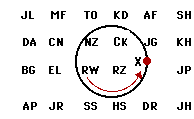6. A physics teacher ties an eraser to the end of a string and then whirls it in a counter-clockwise circle. If the teacher lets go of the string, then the eraser hits a student (or several students) in the classroom. If the string is let go when the eraser is at point X on the diagram at the right, then which student(s) in the class will the eraser hit? Write the initials in this space: ________________

 Answer: JG and AF Once the centripetal force is no longer present, the eraser will follow its straight-line, inertial path. This path would be directed tangent to the circle and would pass through the locations where JG and AF are sitting. View a reasonably cool animation.

 Useful Web Links Speed and Velocity

[ #1 | #2 | #3 | #4 | #5 | #6 | #7 | #8 | #9 | #10 | #11 | #12 | #13 | #14 | #15 | #16 | #17 | #18 | #19 | #20 | #21 | #22 | #23 | #24 | #25 | #26 | #27 | #28 | #29 | #30 | #31 | #32 | #33 | #34 | #35 | #36 | #37 | #38 | #39 | #40 ]

7. Which of the following statements are true about gravitational force? Identify all that apply.

1. The gravitational force only acts between very, very massive objects.
2. The gravitational force between an object and the earth is inversely related to the distance between the object's and the earth's center.
3. The gravitational force can ALWAYS be accurately calculated by multiplying the object mass by the acceleration of gravity (m•g).
4. The gravitational force acting upon an object is the same as the weight of the object.
5. The gravitational force between two objects is independent of the mass of the smaller of the two objects.
6. If object A gravitationally attracts object B with a force of X Newtons, then object B will also gravitationally attract object A with the same force of X Newtons.
7. The doubling of the separation distance (measured from the center) between two objects will halve the gravitational force between the objects.
8. It an object is placed two earth-radii above the surface of the earth, then the force of gravitational attraction between the object and the earth will be one-fourth the magnitude as on earth's surface.
9. Orbiting astronauts do not experience a force of gravity; this explains why they feel weightless.

 Answer: BCDF A is false; gravitational forces are universal (Newton's big idea); they act between any two objects which have mass. B is true; if the distance is increased, then the force is decreased. C is true; this is always the case. It is not true however to say that the gravitational force is equal to mass•9.8 m/s/s. The value of g varies with location and so at distances significantly further from the earth's surface, g is reduced and the gravitational force must be computed using a different value of g. D is true; weight and gravitational force are synonymous. E is false; gravitational force is dependent upon the product of the two masses. Both masses are important in the computation. F is true; for every action there is an equal and opposite reaction. This is Newton's third law, an inescapable reality about forces. G is false; doubling the separation distance will make the force one-fourth the size. H is false; placing the object at two earth-radii above earth's surface will put 3-earth radii from earth's center. This is equivalent to increasing the separation distance by a factor of 3; this decreases the force by a factor of 9. I is false; orbiting astronauts must be experiencing the force of gravity. Without this force, there would be no centripetal force and no orbit.

 Useful Web Links Newton's Law of Universal Gravitation

[ #1 | #2 | #3 | #4 | #5 | #6 | #7 | #8 | #9 | #10 | #11 | #12 | #13 | #14 | #15 | #16 | #17 | #18 | #19 | #20 | #21 | #22 | #23 | #24 | #25 | #26 | #27 | #28 | #29 | #30 | #31 | #32 | #33 | #34 | #35 | #36 | #37 | #38 | #39 | #40 ]

8. Which of the following statements are true about the acceleration of gravity? Identify all that apply.

1. The acceleration of gravity experienced by objects located near to (and far from) from the earth depends upon the mass of the object.
2. The acceleration of gravity experienced by objects located near to (and far from) from the earth depends upon the mass of the Earth.
3. The acceleration of gravity experienced by objects located near to (and far from) the earth is inversely related to the distance between the center of the object and the center of the earth.
4. Increasing the mass of an object will increase the acceleration of gravity experienced by the object.
5. Doubling the distance between an object and the earth's center will decrease the acceleration of gravity by a factor of four.
6. The acceleration of an orbiting satellite is equal to the acceleration of gravity at that particular location.
7. If the mass of the Earth were doubled (without an alteration in its radius), then the acceleration of gravity on its surface would be approximately 20 m/s2.
8. If the mass of the Earth were doubled and the radius of the earth were doubled, then the two changes would offset each other and the acceleration of gravity on its surface would still be approximately 10 m/s2.

 Answer: BCEFG A is false; check out the equation - g = GMcentral/R2. The value of g does not depend upon the object's mass. B is true; check out the equation again. The acceleration of gravity created by the earth depends upon the earth's mass. C is true; check out the equation one more time. The separation distance is located in the denominator of the equation, indicating an inverse relationship. D is false; like statement A, this statement makes a claim that the acceleration of gravity depends upon the mass of the object. It does not. In the derivation of g from the Universal Gravitation equation, the mass cancels. :) E is true; g is inversely proportional to the square of the distance; a doubling of the distance means that you must divide the force of gravity value by 4 (22) to obtain the new force of gravity value. F is true; the acceleration of gravity is the acceleration which is caused by gravity when it is the only force. For an orbiting satellite, gravity is the only force. G is true; according to the equation, the g value is directly proportional to the mass of the earth. An increase in M results in a proportional increase in g. H is false; g is approximately 10 m/s/s on earth's surface. Doubling the mass of the earth would increase g to approximately 20 m/s/s. Then doubling the distance from the surface of the earth to its center would decrease g by a factor of 4. The new acceleration of gravity value would be approximately 5 m/s/s. Clearly, these two alterations do not offset each other.

 Useful Web Links The Value of g

[ #1 | #2 | #3 | #4 | #5 | #6 | #7 | #8 | #9 | #10 | #11 | #12 | #13 | #14 | #15 | #16 | #17 | #18 | #19 | #20 | #21 | #22 | #23 | #24 | #25 | #26 | #27 | #28 | #29 | #30 | #31 | #32 | #33 | #34 | #35 | #36 | #37 | #38 | #39 | #40 ]

9. Which of the following statements are true about satellites? Identify all that apply.

1. Satellites are falling projectiles.
2. All satellites follow circular paths.
3. The orbital velocity required of a satellite is dependent upon the mass of the satellite; a more massive satellite would require a greater orbital speed.
4. The orbital velocity of a satellite does not depend upon the mass of the planet around which it orbits.
5. A high-altitude satellite will require a greater orbital speed than a low-altitude satellite.
6. By definition, a geosynchronous satellite orbits the earth in a perfect circle, maintaining the same distance above the surface of the earth.
7. Satellites travel faster along their orbital path when they are closest to the earth.
8. The acceleration of a satellite varies inversely with its distance from the center of the earth. More distant satellites have smaller accelerations.

 Answer: AGH A is true (mostly); satellites are projectiles which are falling towards the Earth without falling into the Earth.  They are falling in the sense that (like any projectile), they are dropping below their tangential direction of motion. B is false; some satellites travel in elliptical and even very elliptical orbits.  For instance, the planets orbit the Sun in mildly elliptical paths. C is false; the equation for the orbital velocity of a satellite is v = SQRT(G•Mcentral/R).  The Mcentral is the mass of the central body - the body being orbited by the satellite. As seen in the equation, the orbital velocity is independent of the mass of the satellite. D is false; the equation for the orbital velocity of a satellite is v = SQRT(G•Mcentral/R).  The Mcentral is the mass of the central body - the body being orbited by the satellite. Clearly the orbital velocity depends upon the mass of the planet being orbited. E is false the equation for the orbital velocity of a satellite is v = SQRT(G•Mcentral/R).  The R in the denominator inside the radical is the radius of the orbit. The higher altitude satellites have a greaer radius of orbit. As the R in the denominator increases, the required orbital velocity will decrease. F is false; by definition, a geosynchronous satellite has synchronized or matched its orbital period to the rotational period of the Earth about its axis - 24 hours. G is true; this is an idea known since Kepler that is explained by Newton's gravitational law.  As a satellite orbits in an elliptical path, the force of gravitation is greatest at the times when it is closest to the Earth;  this force causes a greater acceleration and speed of the satellite along these nearest locations of its orbit. H is true; the acceleration of a satellite is given by the equation g=G•Mcentral/R2 where R is the radius of the orbit.  The larger the R value, the smaller the acceleration.  This is an inverse square relationship.

 Useful Web Links Circular Motion Principles for Satellites || Mathematics of Satellite Motion

[ #1 | #2 | #3 | #4 | #5 | #6 | #7 | #8 | #9 | #10 | #11 | #12 | #13 | #14 | #15 | #16 | #17 | #18 | #19 | #20 | #21 | #22 | #23 | #24 | #25 | #26 | #27 | #28 | #29 | #30 | #31 | #32 | #33 | #34 | #35 | #36 | #37 | #38 | #39 | #40 ]

10. Which of the following statements are true about the motion of planets about the sun? Identify all that apply.

1. The force of gravity is the only force which acts upon the planets.
2. Their trajectories are highly elliptical.
3. The planets which are furthest from the sun have the greatest period.
4. For any given planet, the speed is greatest when the planet is closest to the sun.
5. The velocity vector is directed tangent to the elliptical path.
6. The net force vector is at all times directed perpendicular to the velocity vector.
7. To keep the planet from escaping the sun's gravitational field, the net force vector is greatest when the planet is furthest from the sun.

 Answer: ACDE A is true; gravity is the only force acting upon a planet. Think about it - what other physical push or pull can you identify as acting upon the earth. (People pushing on it and pulling it don't count.) B is false; their trajectories are indeed elliptical. Yet in most cases, the ellipses are very close to a circle since the eccentricities are very close to 0. C is true; this is in accord with Kepler's third law: T2/R3 = constant. Expressing this equation another way depicts the direct relationship between T and R: T2 = constant • R3. D is true; this is Kepler's second law of planetary motion. In order to sweep out equal areas in equal times, a satellite must be moving faster when closest to the earth. See a clever animation. E is true; at all times during the orbital path, the velocity of the planet is tangent to the path. Since the path has a slightly elliptical nature to it, the force and acceleration vector are not necessarily perpendicular to this velocity vector. The perpendicular orientation of the F and a vectors relative to the v vector is unique to circular motion. An excellent and informative graphic about this can be found online. F is false; see above explanation and the link to the informative graphic. G is false; the net force is a gravitational force. Gravitational forces vary inversely with distance. Thus, a planet will experience less force when furthest from the sun. You might really need to view the informative graphic.

 Useful Web Links Kepler's Three Laws || Circular Motion Principles for Satellites || Mathematics of Satellite Motion

[ #1 | #2 | #3 | #4 | #5 | #6 | #7 | #8 | #9 | #10 | #11 | #12 | #13 | #14 | #15 | #16 | #17 | #18 | #19 | #20 | #21 | #22 | #23 | #24 | #25 | #26 | #27 | #28 | #29 | #30 | #31 | #32 | #33 | #34 | #35 | #36 | #37 | #38 | #39 | #40 ]

11. Explain how something can be moving at a constant speed yet be accelerating at the same time.

 Answer: The object would have to be traveling in a curved path since it is accelerating. Acceleration is defined as a change in velocity over a change in time. If the speed is staying the same, then the velocity must be changing by altering the direction in which the object is heading. A force is required to do this. See an informative animation and further explanation.

[ #1 | #2 | #3 | #4 | #5 | #6 | #7 | #8 | #9 | #10 | #11 | #12 | #13 | #14 | #15 | #16 | #17 | #18 | #19 | #20 | #21 | #22 | #23 | #24 | #25 | #26 | #27 | #28 | #29 | #30 | #31 | #32 | #33 | #34 | #35 | #36 | #37 | #38 | #39 | #40 ]

12. How did Newton come up with the idea that the moon is actually "falling" toward the Earth.

 Answer: Newton made the connection between objects falling (accelerating) towards the earth and objects in space which are accelerating towards the earth while they are in circular motion around the earth. Both are being pulled by the earth due to the gravitational force. The moon stays in orbit due to it having the appropriate tangential velocity that keeps it from coming closer to the earth's surface. The moon, however, is still accelerating at the rate any object would have at that distance from the earth. In a sense, the moon is falling around the earth rather than into the earth.

 Useful Web Links The Apple, the Moon, and the Inverse Square Law

[ #1 | #2 | #3 | #4 | #5 | #6 | #7 | #8 | #9 | #10 | #11 | #12 | #13 | #14 | #15 | #16 | #17 | #18 | #19 | #20 | #21 | #22 | #23 | #24 | #25 | #26 | #27 | #28 | #29 | #30 | #31 | #32 | #33 | #34 | #35 | #36 | #37 | #38 | #39 | #40 ]

13. Distinguish between true- and apparent-weightlessness.

 Answer: True weightlessness occurs when there is an absence of gravitational force on an object. This is not a likely occurrence since every mass in the universe is attracted to every other mass in the universe with some force of gravity. All objects would experience some degree of gravitational attraction (though it may be considerably small). Apparent weightlessness is the sensation of not experiencing any external contact forces. This occurs when an object is in free-fall. Even though the object may have a large force on it, it wouldn't register on a scale that was in free-fall with it. Astronauts appear this way on the space shuttle.

[ #1 | #2 | #3 | #4 | #5 | #6 | #7 | #8 | #9 | #10 | #11 | #12 | #13 | #14 | #15 | #16 | #17 | #18 | #19 | #20 | #21 | #22 | #23 | #24 | #25 | #26 | #27 | #28 | #29 | #30 | #31 | #32 | #33 | #34 | #35 | #36 | #37 | #38 | #39 | #40 ]

14. Describe the apparent weight of a person in an elevator while upward, accelerating downward, and not accelerating.

 Answer: When one stands upright on a scale, the scale measures the force of the scale pushing upwards on the body. As such, the scale does not measure one's true weight, but one's apparent weight. An elevator accelerating someone up must not only apply a force to provide the acceleration but also oppose the gravitational force on the person. Therefore, a scale would register a number larger than the gravitational force if the person is accelerating upward. In this case, a person would experience an apparent weight which is greater than usual. Likewise, if the person is accelerating downward the scale reading must be less than the gravitational force since the gravitational force is accelerating them downward. As such, the person's apparent weight is less than what they are accustomed to experiencing. If the elevator is moving at constant velocity or at rest, the scale reading must match the gravitational force in order to have a net force of 0. The apparent weight would be equal to the actual weight of the person.

[ #1 | #2 | #3 | #4 | #5 | #6 | #7 | #8 | #9 | #10 | #11 | #12 | #13 | #14 | #15 | #16 | #17 | #18 | #19 | #20 | #21 | #22 | #23 | #24 | #25 | #26 | #27 | #28 | #29 | #30 | #31 | #32 | #33 | #34 | #35 | #36 | #37 | #38 | #39 | #40 ]

### Part C: Diagramming and Analysis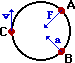15. In the diagram at the right, draw vector arrows (straight lines with arrowheads) which indicate the following for an object which is moving in a clockwise circle.

• the net force at point A.
• the acceleration at point B.
• the velocity at point C.

 Answer: See diagram for answers. The force and acceleration vectors are directed inwards towards the center of the circle and the velocity vector is directed tangent to the circle.

 Useful Web Links Speed and Velocity || Acceleration || The Centripetal Force Requirement

[ #1 | #2 | #3 | #4 | #5 | #6 | #7 | #8 | #9 | #10 | #11 | #12 | #13 | #14 | #15 | #16 | #17 | #18 | #19 | #20 | #21 | #22 | #23 | #24 | #25 | #26 | #27 | #28 | #29 | #30 | #31 | #32 | #33 | #34 | #35 | #36 | #37 | #38 | #39 | #40 ]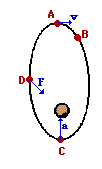16. The diagram at the right shows a satellite orbiting the Earth in an elliptical path in a clockwise direction.

1. Draw a vector representing the velocity of the object at position A.
2. raw a vector representing the force on the object at position D.
3. Draw a vector representing the acceleration of the object at position C.
4. At which of the four positions is the satellite moving fastest?

 Answer: See diagram for answers. The force and acceleration vectors are directed towards the planet being orbited (which is at a foci of the ellipse) and the velocity vector is directed tangent to the path.

 Useful Web Links Circular Motion Principles for Satellites || Mathematics of Satellite Motion

[ #1 | #2 | #3 | #4 | #5 | #6 | #7 | #8 | #9 | #10 | #11 | #12 | #13 | #14 | #15 | #16 | #17 | #18 | #19 | #20 | #21 | #22 | #23 | #24 | #25 | #26 | #27 | #28 | #29 | #30 | #31 | #32 | #33 | #34 | #35 | #36 | #37 | #38 | #39 | #40 ]

17. A 1.20-kg bucket is held by a string and whirled in a vertical circle. The radius of the circle is 1.30 m. The speed of the bucket is 3.70 m/s at the top of the loop and 7.10 m/s at the bottom of the loop. On the diagrams below, construct a free-body diagram (label all forces according to type) for the bucket at both the top and the bottom of the loop. Fill in the blanks and clearly indicate the magnitude of the individual force values on your free-body diagram.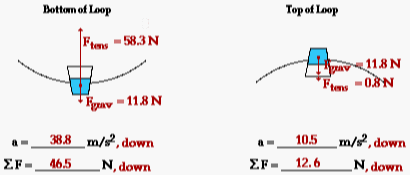Answers: See diagram above. In each case, the acceleration is found using the equation: a = v2/R. For the top of the loop, a = (3.70)2/(1.30) = 10.53 m/s2. For the bottom of the loop, a = (7.10)2/(1.30) = 38.78 m/s2. The net force is always found by Fnet = m•a. At the bottom of the loop, Fnet = (1.20 kg)•(10.53 m/s2) = 12.6 N. At the bottom of the loop, Fnet = (1.20 kg)•(38.78 m/s2) = 46.5 N. The force of gravity is always found by m•g where g = 9.8 m/s2. At both top and bottom of the loop, this value is 11.8 N. From the free-body diagram, one can reason the following: Top: Fnet = Fgrav + Ftens which can be rearranged to Ftens = Fnet - Fgrav Bottom: Fnet = Ftens - Fgrav which can be rearranged to Ftens = Fnet + Fgrav Using these equations and the calculated values of Fgrav and Fnet, one can determine the tension at the top and bottom of the circle.

 Useful Web Links Newton's Second Law - Revisited

[ #1 | #2 | #3 | #4 | #5 | #6 | #7 | #8 | #9 | #10 | #11 | #12 | #13 | #14 | #15 | #16 | #17 | #18 | #19 | #20 | #21 | #22 | #23 | #24 | #25 | #26 | #27 | #28 | #29 | #30 | #31 | #32 | #33 | #34 | #35 | #36 | #37 | #38 | #39 | #40 ]

18. Construct a free-body diagram showing the direction and types of forces acting upon the car at the top and the bottom of a loop. Be sure to label the forces according to type.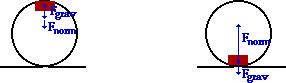Anna experiences a downward acceleration of 24.0 m/s2 at the top of the loop and an upwards acceleration of 12.0 m/s2 at the bottom of the loop. Use Newton's second law to fill in all the blanks and to ultimately determine the normal force acting upon Anna's 800. kg roller coaster car. PSYW

 Top Bottom Fnet = 19200 N, down Fnet = 9600 N, up Fgrav = 7840 N, down Fgrav = 7840 N, down Fnorm = 11360 N, down Fnorm = 17440 N, up

 Answer: See diagram and table above. This problem is very similar to question #17 above. The net force is found by calculating m•a; its direction is in the same direction as the a vector. The gravitational force is found from m•g; the value of 9.8 m/s/s is used for g. Now the free-body diagram can be used to generate the following equations for the top and the bottom of the loop: Top: Fnet = Fgrav + Fnorm which can be rearranged to Fnorm = Fnet - Fgrav Bottom: Fnet = Fnorm - Fgrav which can be rearranged to Fnorm = Fnet + Fgrav The difference in these two equations is attributed to the fact that at the top of the loop, the Fnorm and the Fgrav point in the same direction as the net force vector; at the bottom of the loop, they point in opposite directions. Using the calculated values of Fnet and Fgrav, the normal force can now be calculated.

 Useful Web Links Roller Coasters and Amusement Park Physics

[ #1 | #2 | #3 | #4 | #5 | #6 | #7 | #8 | #9 | #10 | #11 | #12 | #13 | #14 | #15 | #16 | #17 | #18 | #19 | #20 | #21 | #22 | #23 | #24 | #25 | #26 | #27 | #28 | #29 | #30 | #31 | #32 | #33 | #34 | #35 | #36 | #37 | #38 | #39 | #40 ]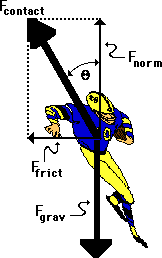19. A fullback is running a sweep around the left side of the line. As he rounds the turn, he is momentarily moving in circular motion, sweeping out a quarter-circle with a radius of 4.17 meters. If the 83.5-kg fullback makes the turn with a speed of 5.21 m/s, then what is his acceleration, the net force, the angle of lean (measured to the vertical), and the total contact force with the ground? PSYW

a = 6.51 m/s2

Fnet = 544 N

Fgrav = 818 N

Fnorm = 818 N

Ffrict = 544 N

Fcontact = 982 N

Angle of Lean = 33.6 degrees

 Answer: See table above, The acceleration is found using the equation: a = v2/R. For the top of the loop, a = (5.21)2/(4.17) = 6.51 m/s2. The net force is always found by Fnet = m•a. For the fullback, Fnet = (83.5 kg)•(6.51 m/s2) = 544 N. This net force is directed inwards and as such, it is equal to the force of friction supplied by the ground. Subsequently, Ffrict = 544 N. The force of gravity is always found by m•g where g = 9.8 m/s2. Using the fullback's mass, this value is 818 N. Since the normal force must support the fullback's weight, it is equal in magnitude to the force of gravity; so Fnorm = 818 N. The contact force is the resultant force of the Fnorm and Ffrict. Its value can be determined using the Pythagorean theorem: Fcontact = SQRT[(544 N)2 + (818 N)2] = 982 N. The angle of lean (theta in the diagram above) can be found using either the sine or the tangent function. From the diagram, it is seen that the sine(theta) = Ffrict /Fcontact. Thus, theta is equal to sin-1(Ffrict /Fcontact) = sin-1 (544 N/982 N) = 33.6 degrees.

[ #1 | #2 | #3 | #4 | #5 | #6 | #7 | #8 | #9 | #10 | #11 | #12 | #13 | #14 | #15 | #16 | #17 | #18 | #19 | #20 | #21 | #22 | #23 | #24 | #25 | #26 | #27 | #28 | #29 | #30 | #31 | #32 | #33 | #34 | #35 | #36 | #37 | #38 | #39 | #40 ]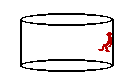20. Pete Zaria is riding the Cliff Hanger at Great America. Pete enters a cylindrical barrel which makes 18.5 revolutions every minute. The diameter of the barrel is 6.92 m. Pete's mass is 64.7 kg. Construct a free-body diagram showing the forces acting upon Pete. Calculate the acceleration and net force acting upon Pete Zaria. Finally, determine the coefficient of friction between Pete and the walls that would be required to support Pete's weight. PSYW

 Answer: 0.755 As usual, Fgrav = m•g = (64.7 kg)•(9.8 m/s/s) = 634 N. This downward force must be balanced by the friction force since there is no vertical acceleration. Therefore, Ffrict = 634 N. The net inward force is supplied by the normal force; so a calculation of the net force will yield the value of the normal force. Acceleration is found from speed and radius. The speed is: v = (18.5 rev/60 sec)• (2 • pi • 3.46 m/rev) = 6.703 m/s. Now the acceleration is: a = v2/R = (6.703 m/s)2/(3.46 m) = 12.986 m/s2 Now the net force (and the normal force) can be calculated: Fnet = Fnorm = m•a = (64.7 kg)•(12.986 m/s/s) = 840.20 N Now that the normal force and the friction force have been found, the coefficient of friction can be determined: µ = (Ffrict)/(Fnorm) = (634 N)/(840.20 N) = 0.755

 Useful Web Links Roller Coasters and Amusement Park Physics

[ #1 | #2 | #3 | #4 | #5 | #6 | #7 | #8 | #9 | #10 | #11 | #12 | #13 | #14 | #15 | #16 | #17 | #18 | #19 | #20 | #21 | #22 | #23 | #24 | #25 | #26 | #27 | #28 | #29 | #30 | #31 | #32 | #33 | #34 | #35 | #36 | #37 | #38 | #39 | #40 ]

### Part D: Short Computations

21. Two objects attract each other with a force of gravity (Fgrav) of 36 N. If the distance separating the objects is doubled, then what is the new force of gravitational attraction? PSYW

22. Two objects attract each other with a force of gravity (Fgrav) of 36 N. If the distance separating the objects is doubled and the masses one of the objects is tripled, then what is the new force of gravitational attraction? PSYW

23. Two objects attract each other with a force of gravity (Fgrav) of 36 N. If the distance separating the objects is increased by a factor of 4 and the masses of both objects are tripled, then what is the new force of gravitational attraction? PSYW

 Answers: For Questions #21-#23, Newton's universal gravitation equation must be used as a guide to thinking about how an alteration in one variable would effect another variable. From the equation, it can be deduced that a change in either one of the masses would produce a proportional change in the force of gravity. However, a change in the separation distance will produce the inverse square effect upon the force of gravity. So increasing separation distance by a factor of x, will decrease the force of gravity by a factor of x2. This reasoning leads to the following solutions. 21. New force = 36 N / 4 = 9.0 N 22. New force = 36 N • 3 / 4 = 27.0 N 23. New force = 36 N •3 •3 / 16 = 20.3 N

 Useful Web Links For Questions #21-#23:  Newton's Law of Universal Gravitation

[ #1 | #2 | #3 | #4 | #5 | #6 | #7 | #8 | #9 | #10 | #11 | #12 | #13 | #14 | #15 | #16 | #17 | #18 | #19 | #20 | #21 | #22 | #23 | #24 | #25 | #26 | #27 | #28 | #29 | #30 | #31 | #32 | #33 | #34 | #35 | #36 | #37 | #38 | #39 | #40 ]

24. Suppose that the acceleration of gravity on the surface of planet X is 12 m/s/s. Determine the acceleration of gravity at a location ...

1. of 2 radii from the center of planet X.
2. of 4 radii from the center of planet X.
3. of 2 radii from the surface of planet X.
4. on the surface of planet X if the planet mass were twice as large (same radius).
5. on the surface of planet X if the planet mass were one-half as large (same radius).

 Answers: Like the force of gravity, the acceleration of gravity varies inversely with distance. So increasing the separation distance by a factor of x, decreases the g value by a factor x2. An object on the surface of planet X is a distance of one-radius from its center.  Success on this question depends on the ability to recognize from the given information the factor X by which the distance is changed (and in part e, the factor by which the mass is changed). 24a. g = (12 m/s/s) / 4 = 3 m/s/s 24b. g = (12 m/s/s) / 16 = 0.75 m/s/s 24c. g = (12 m/s/s) / 9 = 1.33 m/s/s (A distance of 2 radii from the surface is equivalent to 3 radii from the center of the planet.) 24d. g = (12 m/s/s)• 2 = 24 m/s/s 24e. g = (12 m/s/s) • 0.5 = 6 m/s/s

 Useful Web Links The Value of g

[ #1 | #2 | #3 | #4 | #5 | #6 | #7 | #8 | #9 | #10 | #11 | #12 | #13 | #14 | #15 | #16 | #17 | #18 | #19 | #20 | #21 | #22 | #23 | #24 | #25 | #26 | #27 | #28 | #29 | #30 | #31 | #32 | #33 | #34 | #35 | #36 | #37 | #38 | #39 | #40 ]

25. Suppose that a planet was located 12.0 times further from the sun than the earth's distance from the sun. Determine the period of the planet. PSYW

 Answer: 41.6 yr This question explores the R-T relationship. As such, Kepler's third law - that the T2/R3 ratio is the same for all the planets - must be utilized. Tearth2/Rearth3 = Tplanet2/Rplanet3 (1 year)2/Rearth3 = Tplanet2 /(12.0•Rearth)3 Rearranging this equation to solve for the period of the planet yields the equation: Tplanet2 = (1 year)2 • (12.0•Rearth)3 /Rearth3 = (1 year)2 • (12.0)3 = 1728 year2 Tplanet = SQRT(1728 year2 ) = 41.6 years

 Useful Web Links Kepler's Three Laws

[ #1 | #2 | #3 | #4 | #5 | #6 | #7 | #8 | #9 | #10 | #11 | #12 | #13 | #14 | #15 | #16 | #17 | #18 | #19 | #20 | #21 | #22 | #23 | #24 | #25 | #26 | #27 | #28 | #29 | #30 | #31 | #32 | #33 | #34 | #35 | #36 | #37 | #38 | #39 | #40 ]

26. The acceleration of gravity on the moon is approximately one-sixth the value on earth's surface. If a person weighs 60.0 N on the moon's surface, what is his/her approximate mass on Earth? PSYW

 Answer: 36.7 kg If the g value on the moon is 1/6-th of the value of earth (gearth = 9.8 m/s2), then gmoon = 1.63 m/s/s. The mass of a 60.0-N person (on the moon) can then be found: m = Fgrav/g = (60.0 N) / (1.63 m/s/s) = 36.7 kg Since mass is unaffected by location (moon or earth or free space), the mass of the person on earth is the same as the mass on the moon - 36.7 kg.

 Useful Web Links The Value of g

[ #1 | #2 | #3 | #4 | #5 | #6 | #7 | #8 | #9 | #10 | #11 | #12 | #13 | #14 | #15 | #16 | #17 | #18 | #19 | #20 | #21 | #22 | #23 | #24 | #25 | #26 | #27 | #28 | #29 | #30 | #31 | #32 | #33 | #34 | #35 | #36 | #37 | #38 | #39 | #40 ]

27. Determine the force of gravitational attraction between a 52.0-kg mother and a 3.0-kg child if their separation distance is 0.50 meters. PSYW

 Answer: 4.2•10-8 N Use Newton's universal gravitation equation: Fgrav = G • m1 • m2 /R2 = (6.67•10-11 N•m2/kg2) • (52.0 kg) • (3.0 kg) /(0.50 m)2 = 4.2•10-8 N

 Useful Web Links Newton's Law of Universal Gravitation

[ #1 | #2 | #3 | #4 | #5 | #6 | #7 | #8 | #9 | #10 | #11 | #12 | #13 | #14 | #15 | #16 | #17 | #18 | #19 | #20 | #21 | #22 | #23 | #24 | #25 | #26 | #27 | #28 | #29 | #30 | #31 | #32 | #33 | #34 | #35 | #36 | #37 | #38 | #39 | #40 ]

28. Use the following information to determine the orbital velocity at treetop level on the surface of the moon. PSYW

Mass of moon = 7.36 x 1022 kg
Radius of Moon = 1.74 x 106 m

 Answer: 1680 m/s The orbital velocity equation is v = SQRT(G•Mcentral/R) = SQRT[ (6.67•10-11 N•m2/kg2) • (7.36•1022 kg) / (1.74•106 m) ] v = SQRT (2.82•106 m2/s2) = 1680 m/s

 Useful Web Links Mathematics of Satellite Motion

[ #1 | #2 | #3 | #4 | #5 | #6 | #7 | #8 | #9 | #10 | #11 | #12 | #13 | #14 | #15 | #16 | #17 | #18 | #19 | #20 | #21 | #22 | #23 | #24 | #25 | #26 | #27 | #28 | #29 | #30 | #31 | #32 | #33 | #34 | #35 | #36 | #37 | #38 | #39 | #40 ]

### Part E: Problem-Solving

29. A roller coaster car loaded with passengers, has a mass of 500. kg; the radius of curvature of the track at the bottom point of a dip is 12.0 m. The vehicle has a speed of 18.0 m/s at this point.

1. In the space below, draw a free-body diagram for the car (label forces according to type).
2. Calculate the acceleration and the net force acting upon the car. PSYW
3. Calculate the force exerted on the vehicle by the track? PSYW

 Answers: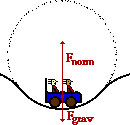The free-body diagram (part a) is shown at the right. The acceleration of the car can be computed as follows: a = v2/R = (18.0 m/s)2/(12.0 m) = 27.0 m/s2 (part b) The net force can be found in the usual manner: Fnet = m•a = (500. kg)•(27.0 m/s2) = 13500 N (part b) Since the center of the circle (see diagram) is above the riders, then both the net force and the acceleration vectors have an upward direction. The force of gravity is downwards, so the net force is equal to the upward force minus the downward force: Fnet = Fnorm - Fgrav where Fgrav = m • g = (500. kg) • (9.8 m/s/s) = 4900 N Thus, Fnorm = Fnet + Fgrav = 13500 N + 4900 N = 18400 N (part c)

 Useful Web Links Roller Coasters and Amusement Park Physics

[ #1 | #2 | #3 | #4 | #5 | #6 | #7 | #8 | #9 | #10 | #11 | #12 | #13 | #14 | #15 | #16 | #17 | #18 | #19 | #20 | #21 | #22 | #23 | #24 | #25 | #26 | #27 | #28 | #29 | #30 | #31 | #32 | #33 | #34 | #35 | #36 | #37 | #38 | #39 | #40 ]

30. What is the acceleration of a piece of dust on an old-fashioned record album, 15 cm from the center, if the record is spinning at 33.3 rpm?

 Answer: 1.8 m/s/s To find acceleration, the speed and the radius must be known. The radius is given; the speed can be computed as distance per time. The dust moves a distance equivalent to 33.3 circumferences in 60 seconds. So v = 33.3 • 2 • pi • (0.15 m) / (60 s) = 0.523 m/s. The acceleration can now be computed using the centripetal acceleration equation: a = v2/R = (0.523 m/s)2 / (0.15 m) = 1.8 m/s/s

 Useful Web Links Mathematics of Circular Motion

[ #1 | #2 | #3 | #4 | #5 | #6 | #7 | #8 | #9 | #10 | #11 | #12 | #13 | #14 | #15 | #16 | #17 | #18 | #19 | #20 | #21 | #22 | #23 | #24 | #25 | #26 | #27 | #28 | #29 | #30 | #31 | #32 | #33 | #34 | #35 | #36 | #37 | #38 | #39 | #40 ]

31. What is the tension in a 0.500 meter rope which carries a 2.50 kg bucket of water in a vertical circle with a velocity of 3.00 m/s when the bucket is at the bottom of its swing?

 Answer: 69.5 N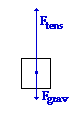Begin with a free-body diagram for the bucket at the bottom of its string. Since the bucket is at the bottom of the circle, the net force is upwards (inwards). Notice that the tension force must be greater than the force of gravity in order to have a net upwards (inwards) force. Also note that while Ftens is directed in the direction of the net force, Fgrav is in the opposite direction. Thus, the equation can be written: Fnet = Ftens - Fgrav This equation can be rearranged to solve for tension; the expressions for Fgrav (m•g) and Fnet (m•v2/R) can be substituted into it: Ftens = Fnet + Fgrav = m•v2/R + m•g = (2.50 kg)•(3.00 m/s)2/(0.500 m) + (2.50 kg)•(9.8 m/s2) Ftens = 69.5 N

 Useful Web Links Newton's Second Law - Revisited

[ #1 | #2 | #3 | #4 | #5 | #6 | #7 | #8 | #9 | #10 | #11 | #12 | #13 | #14 | #15 | #16 | #17 | #18 | #19 | #20 | #21 | #22 | #23 | #24 | #25 | #26 | #27 | #28 | #29 | #30 | #31 | #32 | #33 | #34 | #35 | #36 | #37 | #38 | #39 | #40 ]

32. A 53.5-kg rider on a roller coaster car is moving 10.3 m/s at the top of a loop which has a radius of curvature of 7.29 m. Determine the normal force experienced by the rider.

 Answer: 254 N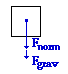At the top of the loop, both the gravitational and the normal force are directed inwards (as shown in the free-body diagram at the right). Thus, the Fnet equation can be written as: Fnet = Fgrav + Fnorm This equation can be rearranged and the expressions for Fgrav (m•g) and Fnet (m•v2/R) can be substituted into it: Fnorm = Fnet - Fgrav = m • v2 / R - m • g Fnorm = (53.5 kg) • (10.3 m/s)2 / (7.29 m) - (53.5 kg) • (9.8 m/s2) Fnorm = 254 N

 Useful Web Links Roller Coasters and Amusement Park Physics

[ #1 | #2 | #3 | #4 | #5 | #6 | #7 | #8 | #9 | #10 | #11 | #12 | #13 | #14 | #15 | #16 | #17 | #18 | #19 | #20 | #21 | #22 | #23 | #24 | #25 | #26 | #27 | #28 | #29 | #30 | #31 | #32 | #33 | #34 | #35 | #36 | #37 | #38 | #39 | #40 ]

33. Determine the minimum coefficient of friction required to keep a 920.-kg car on a turn with a radius of 26.8 m. The car is traveling 29.9 m/s and the roadway is level.

 Answer: 3.40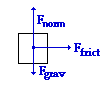As usual, begin with a free-body diagram. The gravity force is balanced by (and equal to) the normal force and the force of friction is the net force. The solution then begins by equating m • a to Ffrict and carrying out the customary substitutions and algebra steps (using the fact that a = v2 / R and Ffrict = µ • Fnorm and Fgrav = m • g). m•a = Ffrict m • v2/R = µ • Fnorm m • v2/R = µ • m • g The mass now cancels and the equation can be rearranged to solve for mu: µ = v2/(g• R) = (29.9 m/s)2 / [ (9.8 m/s2) • (26.8 m)] = 3.40 (This is not a likely mu value and as such the car does not have much of a chance negotiating the turn at this speed.)

 Useful Web Links Newton's Second Law - Revisited

[ #1 | #2 | #3 | #4 | #5 | #6 | #7 | #8 | #9 | #10 | #11 | #12 | #13 | #14 | #15 | #16 | #17 | #18 | #19 | #20 | #21 | #22 | #23 | #24 | #25 | #26 | #27 | #28 | #29 | #30 | #31 | #32 | #33 | #34 | #35 | #36 | #37 | #38 | #39 | #40 ]

34. With what speed (in mi/hr) must you drive your car across the crest of a hill of radius 37.1-m in order to feel weightless (1.00 m/s = 2.24 mi/hr)?

 Answer: 42.8 mi/hr (19.1 m/s) The feeling of weightlessness is experienced when a person is under the sole influence of gravity. So the free-body diagram for such a person would reveal only one force - gravity, pointing straight down. In such a case, the seat does not push upward upon the person. The car has passed over the crest of the hill at such a high speed that it is momentarily raised off the roadway and is unable to support the weight of its passengers. The force of gravity is the net force and the following equation can be written: m • a = m • g The mass can be canceled from both sides of the equation (sigh!) and the expression for acceleration can be substituted into the equation, yielding: v2/R = g Rearrangement and substitution yields the solution: v = SQRT(g • R) = [(9.8 m/s2) • (37.1 m)] = SQRT[364 m2/s2] = 19.1 m/s This value for speed can be converted to mi/hr using the equivalency: 2.24 mi/hr = 1.00  m/s; the car is traveling with a speed of 42.8 mi/hr.

 Useful Web Links Newton's Second law - Revisited || Weightlessness

[ #1 | #2 | #3 | #4 | #5 | #6 | #7 | #8 | #9 | #10 | #11 | #12 | #13 | #14 | #15 | #16 | #17 | #18 | #19 | #20 | #21 | #22 | #23 | #24 | #25 | #26 | #27 | #28 | #29 | #30 | #31 | #32 | #33 | #34 | #35 | #36 | #37 | #38 | #39 | #40 ]

35. What is the reading of a bathroom scale when a man of mass 72 kg stands on it while riding in an elevator accelerating upwards at 5.4 m/s2?

 Answer: 1.1 x 103 N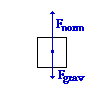The scale reading is a measure of the amount of normal force acting upon the person. As is the usual case, begin with a free-body diagram (shown at the right). Since the acceleration is upwards, the upwards normal force (Fnorm) is greater than the downwards gravitational force (Fgrav). From the diagram, the Fnet equation can be written: Fnet = Fnorm - Fgrav Algebraic rearrangement of this equation, combined with the understanding that Fnet = m•a and Fgrav = m•g yields the following solution: Fnorm = Fnet + Fgrav = m•a + m•g = (72 kg) • (5.4 m/s2) + (72 kg) • (9.8 m/s2) Fnorm = 1.1 x 103 N

[ #1 | #2 | #3 | #4 | #5 | #6 | #7 | #8 | #9 | #10 | #11 | #12 | #13 | #14 | #15 | #16 | #17 | #18 | #19 | #20 | #21 | #22 | #23 | #24 | #25 | #26 | #27 | #28 | #29 | #30 | #31 | #32 | #33 | #34 | #35 | #36 | #37 | #38 | #39 | #40 ]

36. An airplane pilot makes a vertical loop with a 56-2-m radius. Determine the normal force acting upon the pilot's 62.6-kg body at the top of the loop if his air speed is 64.1 m/s.

 Answer: 3.96 x 103 N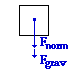As usual, begin with a free-body diagram (shown at the right). From the diagram, the equation relating net force to the individual forces can be written: Fnet = Fnorm + Fgrav Since Fnet = m•a = m•v2/R and Fgrav = m•g, substitutions can be made and the equation can be rewritten: m•v2/R = Fnorm + m•g Rearranging to solve for Fnorm yields: Fnorm = m•v2/R - m•g = (62.6 kg) • (64.1 m/s)2 / (56.2 m) - (62.6 kg) • (9.8 m/s2) Fnorm = 3.96 x 103 N

 Useful Web Links Newton's Second Law - Revisited

[ #1 | #2 | #3 | #4 | #5 | #6 | #7 | #8 | #9 | #10 | #11 | #12 | #13 | #14 | #15 | #16 | #17 | #18 | #19 | #20 | #21 | #22 | #23 | #24 | #25 | #26 | #27 | #28 | #29 | #30 | #31 | #32 | #33 | #34 | #35 | #36 | #37 | #38 | #39 | #40 ]

37. Use the following information to help determine the acceleration of the planet Pluto about the sun.

Mass of Sun = 1.991 x 1030 kg
Sun-Pluto Distance = 5.91 x 1012 m

 Answer: g = 6.56 • 10-6 m/s2 Pluto's acceleration is caused by the gravitational force of attraction to the sun. Thus, one way (there are a couple of ways) to calculate the a of Pluto is to determine the acceleration of gravity towards the sun at the location of Pluto's orbit. The equation, substitution (using textbook values) and solution are shown below: g = G • Msun / R2 = (6.67 • 10-11 N•m2/kg2) • (1.991 • 1030 kg) / (4.50 • 1012 m)2 g = 6.56 • 10-6 m/s2

 Useful Web Links The Value of g

[ #1 | #2 | #3 | #4 | #5 | #6 | #7 | #8 | #9 | #10 | #11 | #12 | #13 | #14 | #15 | #16 | #17 | #18 | #19 | #20 | #21 | #22 | #23 | #24 | #25 | #26 | #27 | #28 | #29 | #30 | #31 | #32 | #33 | #34 | #35 | #36 | #37 | #38 | #39 | #40 ]

38. What is the period of rotation of Venus in earth years if it is a distance of 1.08 x 1011 meters from the sun? (The earth-sun distance is 1.5 x 1011 m.)

 Answer: 0.61 yr This question explores the R-T relationship. As such, Kepler's third law - that the T2/R3 ratio is the same for all the planets - must be utilized. Tearth2/Rearth3 = TVenus2/RVenus3 (1 year)2/(1.5 x 1011)3 = TVenus2 /(1.08 x 1011 m)3 Rearranging this equation to solve for the period of the planet yields the equation: TVenus2 = (1 year)2 • (1.08 x 1011 m)3 /(1.5 x 1011)3 = (1 year)2) • (0.72)3 = 0.373 year2 TVenus = SQRT(0.373 year2 ) = 0.61 years

 Useful Web Links Kepler's Three Laws

[ #1 | #2 | #3 | #4 | #5 | #6 | #7 | #8 | #9 | #10 | #11 | #12 | #13 | #14 | #15 | #16 | #17 | #18 | #19 | #20 | #21 | #22 | #23 | #24 | #25 | #26 | #27 | #28 | #29 | #30 | #31 | #32 | #33 | #34 | #35 | #36 | #37 | #38 | #39 | #40 ]

39. What is the gravitational attraction between the proton and electron in an Hydrogen atom if they are 5.3 x 10-11 meters apart? (mproton = 1.67 x 10-27 kg; melectron = 9.11x10-31 kg)

 Answer: 3.6•10-47 N Use Newton's universal gravitation equation: Fgrav = G • m1 • m2 /R2 Fgrav = (6.67•10-11 N•m2/kg2) • (1.67 x 10-27 kg) • (9.11x10-31 kg) /(5.3 x 10-11 m)2 Fgrav = 3.6•10-47 N

 Useful Web Links Newton's Law of Universal Gravitation

[ #1 | #2 | #3 | #4 | #5 | #6 | #7 | #8 | #9 | #10 | #11 | #12 | #13 | #14 | #15 | #16 | #17 | #18 | #19 | #20 | #21 | #22 | #23 | #24 | #25 | #26 | #27 | #28 | #29 | #30 | #31 | #32 | #33 | #34 | #35 | #36 | #37 | #38 | #39 | #40 ]

40. Use the following information to help determine the orbital velocity and orbital period of a satellite at a location of 15000 miles above the surface of the earth.

Mass of Earth = 5.98 x 1024 kg
Radius of Earth = 6.37 x 106 m
1609 m = 1.00 mi

 Answers: The first step is to find the separation distance from the center of the earth - this is R in most of the equations. The altitude of the satellite is 15000 mi or 2.41•107 m; this distance must be added to the radius of the earth to determine the separation distance. The radius of the earth is 6.38•106 m; the separation distance is 3.05•107 m. The orbital velocity can be computed using the orbital velocity equation: v = SQRT(G•Mcentral/R) = SQRT[ (6.67•10-11 N•m2/kg2) • (5.98•1024 kg) / (3.05•107 m) ] v = SQRT (1.31•107 m2/s2) = 3615 m/s The orbital period can now be calculated using the v = 2•pi•R/T equation or the Kepler's second law equation [ T2/R3 = 4•pi2/(GMearth)]. Starting with the first equation, algebra yields: T = (2•pi•R)/v = [2 • pi • (3.05•107 m) / (3615 m/s) ] = 5.30 • 104 s = 14.7 hr

 Useful Web Links Mathematics of Satellite Motion

[ #1 | #2 | #3 | #4 | #5 | #6 | #7 | #8 | #9 | #10 | #11 | #12 | #13 | #14 | #15 | #16 | #17 | #18 | #19 | #20 | #21 | #22 | #23 | #24 | #25 | #26 | #27 | #28 | #29 | #30 | #31 | #32 | #33 | #34 | #35 | #36 | #37 | #38 | #39 | #40 ]

## You Might Also Like ...

Users of The Review Session are often looking for learning resources that provide them with practice and review opportunities that include built-in feedback and instruction. If that is what you're looking for, then you might also like the following:

The Calculator Pad includes physics word problems organized by topic. Each problem is accompanied by a pop-up answer and an audio file that explains the details of how to approach and solve the problem. It's a perfect resource for those wishing to improve their problem-solving skills.

Visit: The Calculator Pad Home | Calculator Pad - Circular Motion and Gravitation

2. Minds On Physics the App Series

Minds On Physics the App ("MOP the App") is a series of interactive questioning modules for the student that is serious about improving their conceptual understanding of physics. Each module of the series covers a different topic and is further broken down into sub-topics. A "MOP experience" will provide a learner with challenging questions, feedback, and question-specific help in the context of a game-like environment. It is available for phones, tablets, Chromebooks, and Macintosh computers. It's a perfect resource for those wishing to refine their conceptual reasoning abilities. Part 2 of the series includes topics in Circular Motion and Gravitation.

Visit: MOP the App Home || MOP the App - Part 2